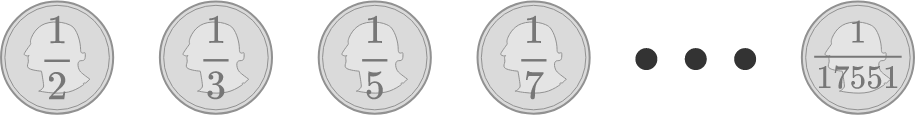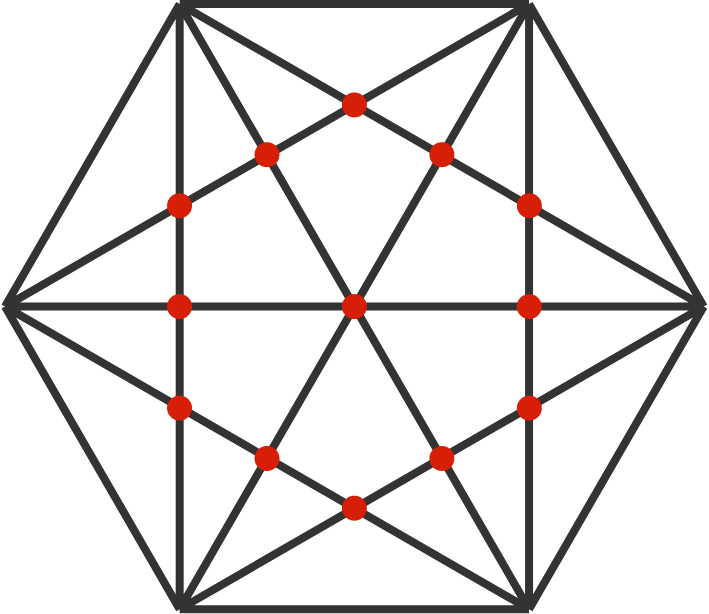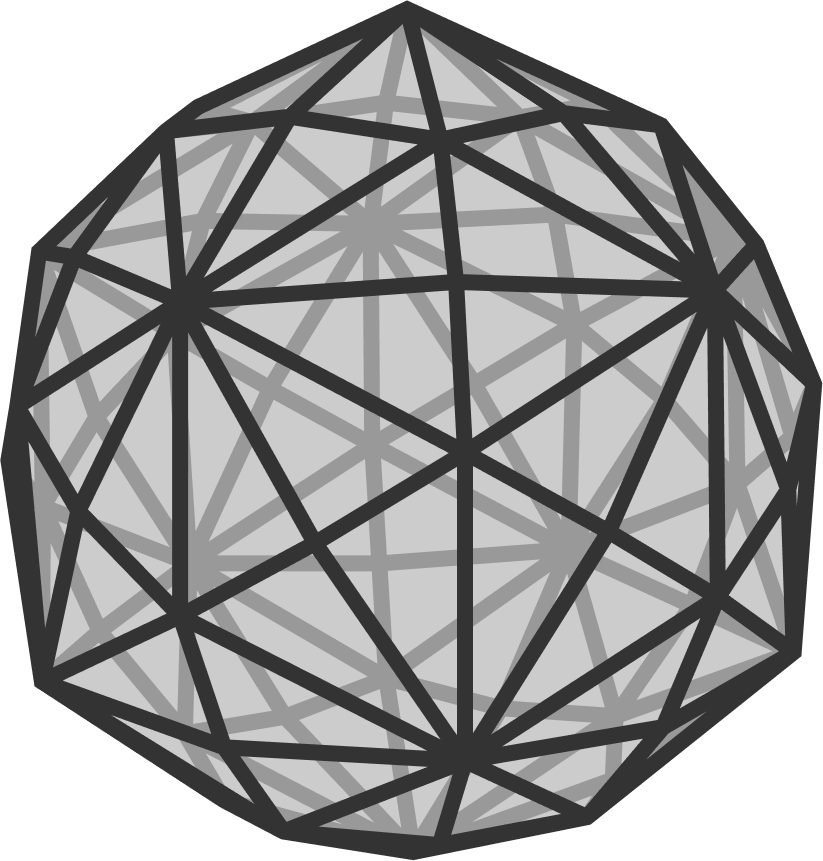# Problems of the Week

Contribute a problem

Ram has 2018 coins.

The $1^\text{st}$ coin has a $\frac{1}{2}$ chance of flipping heads, the $2^\text{nd}$ coin has a $\frac{1}{3}$ chance of flipping heads, the $3^\text{rd}$ coin has a $\frac{1}{5}$ chance of flipping heads, continuing such that the $k^\text{th}$ coin has a $\frac{1}{p(k)}$ chance of flipping heads, where $p(k)$ is the $k^\text{th}$ prime number.

Ram flips each of his coins once.

What is the probability that Ram flips an even number of heads?From a string of length $1 \text{ m},$ we choose a length uniformly at random between $0 \text{ m}$ and $1 \text{ m},$ and cut as many of these lengths as possible from the string.

What is the expected length of the remaining string (in meters)?

Bonus: If we have a random number $a$ chosen with uniform distribution over the interval $\big(0,\frac{1}{n}\big],$ where $n\in\mathbb{N}$, what's the expected value of the remainder $1\bmod a$ for a particular $n?$

For $m>1$, it can be proven that the integer sequence $f_m(n) = \gcd(n+m,mn+1)$ has a fundamental period $T_m.$ In other words, $\forall n \in \mathbb{N}, \space f_m(n+T_m) = f_m(n).$ Find an expression for $T_m$ in terms of $m,$ and then give your answer as $T_{12}.$$f(n)$ is the number of intersections of diagonals inside a regular $n$-sided polygon.

For example, in the diagram, $f(6)=13.$

Which is larger, $f(2017)$ or $f(2018)?$The Brilliant logo is similar in appearance to a Disdyakis triacontahedron, a Catalan solid. Suppose we had a circuit consisting of the edges and vertices of one of these solids, and the resistance along any edge is $1\, \Omega.$

The North and South pole vertices of this solid each have 10 connected edges. Determine the equivalent resistance between the poles of the solid in $\Omega.$

×